×#### Thank you for registering.

One of our academic counsellors will contact you within 1 working day.

Click to Chat

1800-1023-196

+91-120-4616500

CART 0

• 0

MY CART (5)

Use Coupon: CART20 and get 20% off on all online Study Material

ITEM
DETAILS
MRP
DISCOUNT
FINAL PRICE
Total Price: Rs.

There are no items in this cart.
Continue Shopping• Complete JEE Main/Advanced Course and Test Series
• OFFERED PRICE: Rs. 15,900
• View Details

```Chapter 14: Lines and Angles Exercise – 14.1

Question: 1

Write down each pair of adjacent angles shown in FigureSolution:

The angles that have common vertex and a common arm are known as adjacent angles

∠DOC and ∠BOC

∠COB and ∠BOA

Question: 2

In figure, name all the pairs of adjacent angles.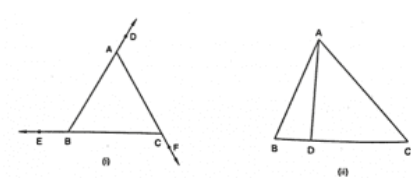Solution:

In fig (i), the adjacent angles are

∠EBA and ∠ABC

∠ACB and ∠BCF

In fig (ii), the adjacent angles are

∠BDA and ∠CDA

Question: 3

In fig , write down

(i) each linear pair

(ii) each pair of vertically opposite angles.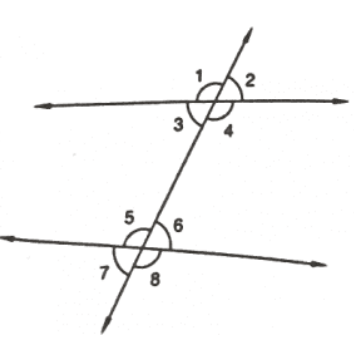Solution:

(i) The two adjacent angles are said to form a linear pair of angles if their non – common arms are two opposite rays.

∠1 and ∠3

∠1 and ∠2

∠4 and ∠3

∠4 and ∠2

∠5 and ∠6

∠5 and ∠7

∠6 and ∠8

∠7 and ∠8

(ii) The two angles formed by two intersecting lines and have no common arms are called vertically opposite angles.

∠1 and ∠4

∠2 and ∠3

∠5 and ∠8

∠6 and ∠7

Question: 4

Are the angles 1 and 2 in figure adjacent angles?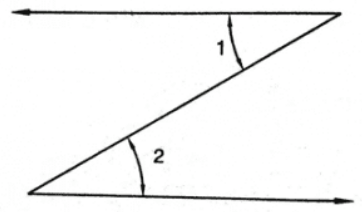Solution:

No, because they do not have common vertex.

Question: 5

Find the complement of each of the following angles:

(i) 35°

(ii) 72°

(iii) 45°

(iv) 85°

Solution:

The two angles are said to be complementary angles if the sum of those angles is 90°

Complementary angles for the following angles are:

(i) 90° – 35° = 55°

(ii) 90° – 72° = 18°

(iii) 90° – 45° = 45°

(iv) 90° – 85° = 5°

Question: 6

Find the supplement of each of the following angles:

(i) 70°

(ii) 120°

(iii) 135°

(iv) 90°

Solution:

The two angles are said to be supplementary angles if the sum of those angles is 180°

(i) 180° – 70° = 110°

(ii) 180° – 120° = 60°

(iii) 180° – 135° = 45°

(iv) 180° – 90° = 90°

Question: 7

Identify the complementary and supplementary pairs of angles from the following pairs

(i) 25°, 65°

(ii) 120°, 60°

(iii) 63°, 27°

(iv) 100°, 80°

Solution:

(i) 25° + 65° = 90° so, this is a complementary pair of angle.

(ii) 120° + 60° = 180° so, this is a supplementary pair of angle.

(iii) 63° + 27° = 90° so, this is a complementary pair of angle.

(iv) 100° + 80° = 180° so, this is a supplementary pair of angle.

Here, (i) and (iii) are complementary pair of angles and (ii) and (iv) are supplementary pair of angles.

Question: 8

Can two obtuse angles be supplementary, if both of them be

(i) obtuse?

(ii) right?

(iii) acute?

Solution:

(i) No, two obtuse angles cannot be supplementary

Because, the sum of two angles is greater than 90 degrees so their sum will be greater than 180degrees.

(ii) Yes, two right angles can be supplementary

Because, 90° + 90° = 180°

(iii) No, two acute angle cannot be supplementary

Because, the sum of two angles is less than 90 degrees so their sum will also be less tha 90 degrees.

Question: 9

Name the four pairs of supplementary angles shown in Fig.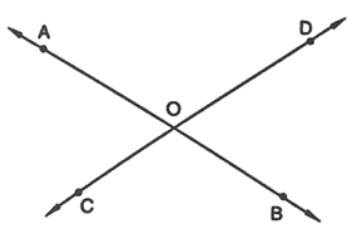Solution:

The supplementary angles are

∠AOC and ∠COB

∠BOC and ∠DOB

∠BOD and ∠DOA

∠AOC and ∠DOA

Question: 10

In Figure, A, B, C are collinear points and ∠DBA = ∠EBA.

(i) Name two linear pairs.

(ii) Name two pairs of supplementary angles.Solution:

(i) Linear pairs

∠ABD and ∠DBC

∠ABE and ∠EBC

Because every linear pair forms supplementary angles, these angles are

∠ABD and ∠DBC

∠ABE and ∠EBC

Question: 11

If two supplementary angles have equal measure, what is the measure of each angle?

Solution:

Let p and q be the two supplementary angles that are equal

∠p = ∠q

So,

∠p + ∠q = 180°

=> ∠p + ∠p = 180°

=> 2∠p = 180°

=> ∠p = 180°/2

=> ∠p = 90°

Therefore, ∠p = ∠q = 90°

Question: 12

If the complement of an angle is 28°, then find the supplement of the angle.

Solution:

Here, let p be the complement of the given angle 28°

Therefore, ∠p + 28° = 90°

=> ∠p = 90° – 28°

= 62°

So, the supplement of the angle = 180° – 62°

= 118°

Question: 13

In Figure, name each linear pair and each pair of vertically opposite angles.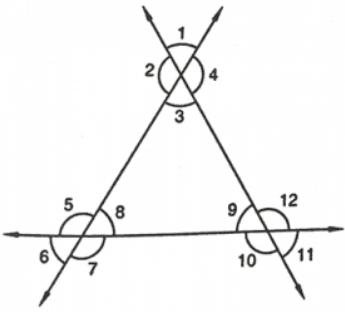Solution:

Two adjacent angles are said to be linear pair of angles, if their non-common arms are two opposite rays.

∠1 and ∠2

∠2 and ∠3

∠3 and ∠4

∠1 and ∠4

∠5 and ∠6

∠6 and ∠7

∠7 and ∠8

∠8 and ∠5

∠9 and ∠10

∠10 and ∠11

∠11 and ∠12

∠12 and ∠9

The two angles are said to be vertically opposite angles if the two intersecting lines have no common arms.

∠1 and ∠3

∠4 and ∠2

∠5 and ∠7

∠6 and ∠8

∠9 and ∠11

∠10 and ∠12

Question: 14

In Figure, OE is the bisector of ∠BOD. If ∠1 = 70°, Find the magnitude of ∠2, ∠3, ∠4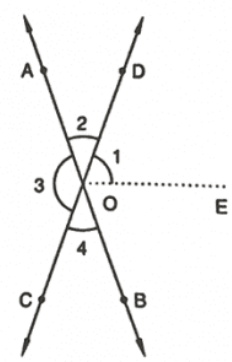Solution:

Given,

∠1 = 70°

∠3 = 2(∠1)

= 2(70°)

= 140°

∠3 = ∠4

As, OE is the angle bisector,

∠DOB = 2(∠1)

= 2(70°)

= 140°

∠DOB + ∠AOC + ∠COB +∠DOB = 360°

=> 140° + 140° + 2(∠COB) = 360°

Since, ∠COB = ∠AOD

=> 2(∠COB) = 360° – 280°

=> 2(∠COB) = 80°

=> ∠COB = 80°/2

=> ∠COB = 40°

Therefore, ∠COB = ∠AOB = 40°

The angles are,

∠1 = 70°,

∠2 = 40°,

∠3 = 140°,

∠4 = 40°

Question: 15

One of the angles forming a linear pair is a right angle. What can you say about its other angle?

Solution:

One of the Angle of a linear pair is the right angle (90°)

Therefore, the other angle is

=> 180° – 90° = 90°

Question: 16

One of the angles forming a linear pair is an obtuse angle. What kind of angle is the other?

Solution:

One of the Angles of a linear pair is obtuse, then the other angle should be acute, only then their sum will be 180°.

Question: 17

One of the angles forming a linear pair is an acute angle. What kind of angle is the other?

Solution:

One of the Angles of a linear pair is acute, then the other angle should be obtuse, only then their sum will be 180°.

Question: 18

Can two acute angles form a linear pair?

Solution:

No, two acute angles cannot form a linear pair because their sum is always less than 180°.

Question: 19

If the supplement of an angle is 65°, then find its complement.

Solution:

Let x be the required angle

So,

=> x + 65° = 180°

=> x = 180° – 65°

= 115°

But the complement of the angle cannot be determined.

Question: 20

Find the value of x in each of the following figures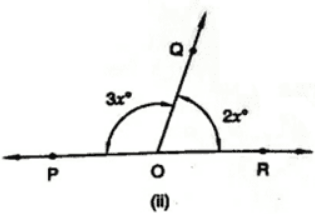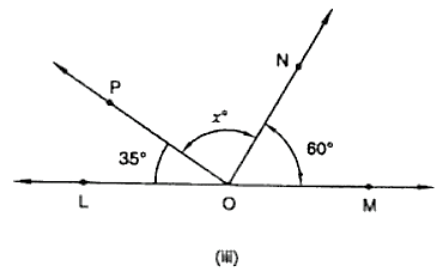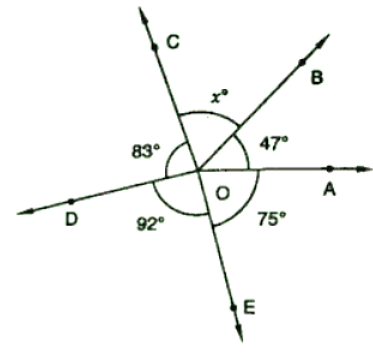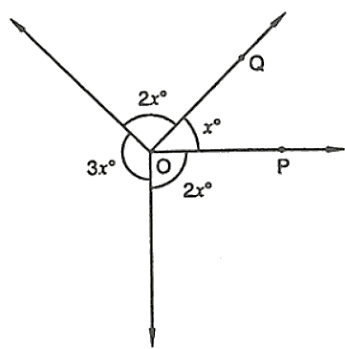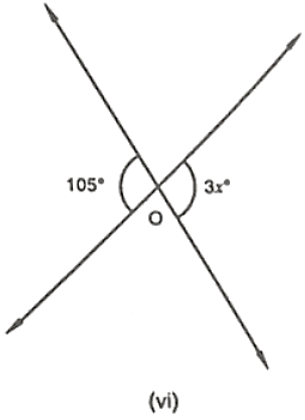Solution:

(i) Since, ∠BOA + ∠BOC = 180°

Linear pair:

=> 60° + x° = 180°

=> x° = 180° – 60°

=> x° = 120°

(ii) Linear pair:

=> 3x° + 2x° = 180°

=> 5x° = 180°

=> x° = 180°/5

=> x° = 36°

(iii) Linear pair,

Since, 35° + x° + 60° = 180°

=> x° = 180° – 35° – 60°

=> x° = 180° – 95°

=> x° = 85°

(iv) Linear pair,

83° + 92° + 47° + 75° + x° = 360°

=> x° + 297° = 360°

=> x° = 360° – 297°

=> x° = 63°

(v) Linear pair,

3x° + 2x° + x° + 2x° = 360°

=> 8x° = 360°

=> x° = 360°/8

=> x∘ = 45°

(vi) Linear pair:

3x° = 105°

=> x° = 105°/3

=> x° = 45°

Question:

In Fig. 22, it being given that ∠1 = 65°, find all the other angles.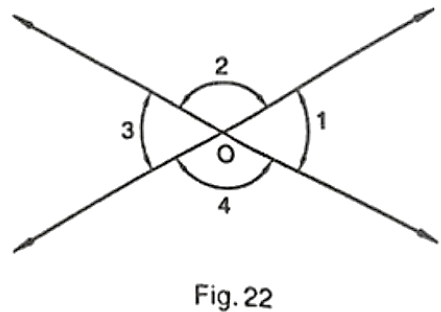Solution:

Given,

∠1 = ∠3 are the vertically opposite angles

Therefore, ∠3 = 65°

Here, ∠1 + ∠2 = 180° are the linear pair

Therefore, ∠2 = 180° – 65°

= 115°

∠2 = ∠4 are the vertically opposite angles

Therefore, ∠2 = ∠4 = 115°

And ∠3 = 65°

Question: 22

In Fig. 23 OA and OB are the opposite rays:

(i) If x = 25°, what is the value of y?

(ii) If y = 35°, what is the value of x?Solution:

∠AOC + ∠BOC = 180° – Linear pair

=> 2y + 5 + 3x = 180°

=> 3x + 2y = 175°

(i) If x = 25°, then

=> 3(25°) + 2y = 175°

=> 75° + 2y = 175°

=> 2y = 175° – 75°

=> 2y = 100°

=> y = 100°/2

=> y = 50°

(ii) If y = 35°, then

3x + 2(35°) = 175°

=> 3x + 70° = 175°

=> 3x = 175° – 70°

=> 3x = 105°

=> x = 105°/3

=> x = 35°

Question: 24

In Figure, write all pairs of adjacent angles and all the linear pairs.Solution:

∠DOA and ∠DOC

∠BOC and ∠COD

∠AOD and ∠BOD

∠AOC and ∠BOC

Linear pairs:

∠AOD and ∠BOD

∠AOC and ∠BOC

Question: 25

In Figure, find ∠x. Further find ∠BOC, ∠COD, ∠AOD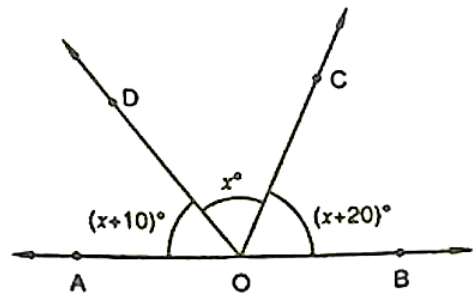Solution:

(x + 10)° + x° + (x + 20) ° = 180°

=> 3x° + 30° = 180°

=> 3x° = 180° – 30°

=> 3x° = 150°

=> x° = 150°/3

=> x° = 50°

Here,

∠BOC = (x + 20)°

= (50 + 20)°

= 70°

∠COD = 50°

∠AOD = (x + 10)°

= (50 + 10)°

= 60°

Question: 25

How many pairs of adjacent angles are formed when two lines intersect in a point?

Solution:

If the two lines intersect at a point, then four adjacent pairs are formed and those are linear.

Question: 26

How many pairs of adjacent angles, in all, can you name in Figure?Solution:

∠EOD and ∠DOC

∠COD and ∠BOC

∠COB and ∠BOA

∠AOB and ∠BOD

∠BOC and ∠COE

∠COD and ∠COA

∠DOE and ∠DOB

∠EOD and ∠DOA

∠EOC and ∠AOC

∠AOB and ∠BOE

Question: 27

In Figure, determine the value of x.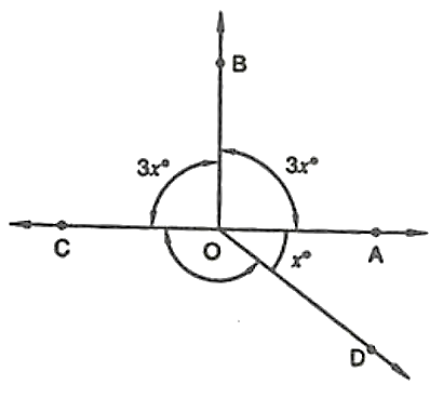Solution:

Linear pair:

∠COB + ∠AOB = 180°

=> 3x° + 3x° = 180°

=> 6x° = 180°

=> x° = 180°/6

=> x° = 30°

Question: 28

In Figure, AOC is a line, find x.Solution:

∠AOB + ∠BOC = 180°

Linear pair

=> 2x + 70° = 180°

=> 2x = 180° – 70°

=> 2x = 110°

=> x = 110°/2

=> x = 55°

Question: 29

In Figure, POS is a line, find x.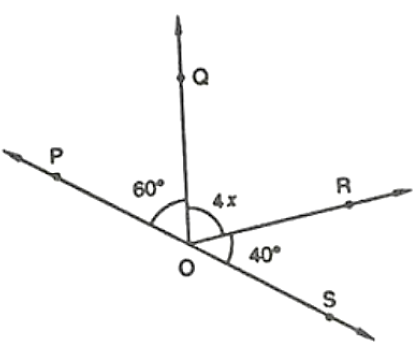Solution:

Angles of a straight line,

∠QOP + ∠QOR + ∠ROS = 108°

=> 60° + 4x + 40° = 180°

=> 100° + 4x = 180°

=> 4x = 180° – 100°

=> 4x = 80°

=> x = 80°/4

=> x = 20°

Question: 30

In Figure, lines l1 and l2 intersect at O, forming angles as shown in the figure. If x = 45°, find the values of y, z and u.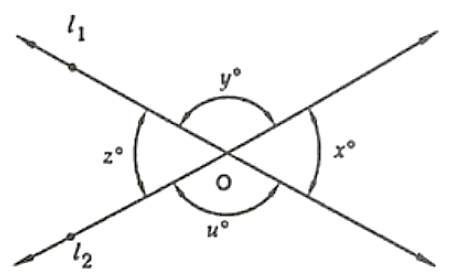Solution:

Given that,

∠x = 45°

∠x = ∠z = 45°

∠y = ∠u

∠x + ∠y + ∠z + ∠u = 360°

=> 45° + 45° + ∠y + ∠u = 360°

=> 90° + ∠y + ∠u = 360°

=> ∠y + ∠u = 360° – 90°

=> ∠y + ∠u = 270°

=> ∠y + ∠z = 270°

=> 2∠z = 270°

=> ∠z = 135°

Therefore, ∠y = ∠u = 135°

So, ∠x = 45°,

∠y = 135°,

∠z = 45°,

∠u = 135°

Question: 31

In Figure, three coplanar lines intersect at a point O, forming angles as shown in the figure. Find the values of x, y, z and u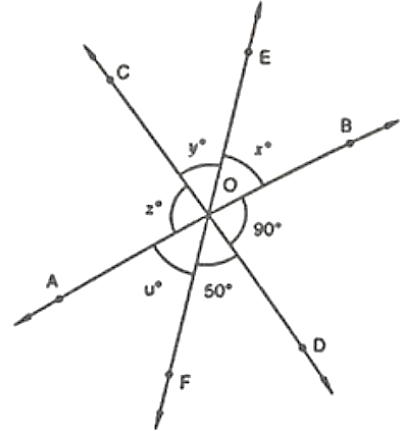Solution:

Given that,

∠x + ∠y + ∠z + ∠u + 50° + 90° = 360°

Linear pair,

∠x + 50° + 90° = 180°

=> ∠x + 140° = 180°

=> ∠x = 180° – 140°

=> ∠x = 40°

∠x = ∠u = 40° are vertically opposite angles

=> ∠z = 90° is a vertically opposite angle

=> ∠y = 50° is a vertically opposite angle

Therefore, ∠x = 40°,

∠y = 50°,

∠z = 90°,

∠u = 40°

Question: 32

In Figure, find the values of x, y and z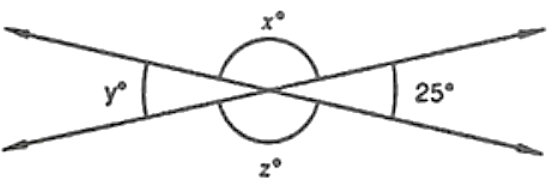Solution:

∠y = 25° vertically opposite angle

∠x = ∠y are vertically opposite angles

∠x + ∠y + ∠z + 25° = 360°

=> ∠x + ∠z + 25° + 25° = 360°

=> ∠x + ∠z + 50° = 360°

=> ∠x + ∠z = 360° – 50°

=> 2∠x = 310°

=> ∠x = 155°

And, ∠x = ∠z = 155°

Therefore, ∠x = 155°,

∠y = 25°,

∠z = 155°

```### Course Features

• 728 Video Lectures
• Revision Notes
• Previous Year Papers
• Mind Map
• Study Planner
• NCERT Solutions
• Discussion Forum
• Test paper with Video Solution